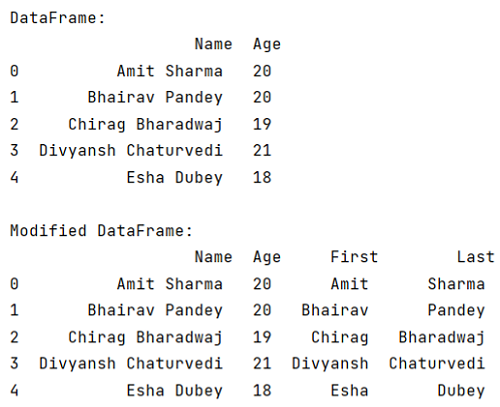# How to split a DataFrame string column into two columns?

Given a DataFrame, we have to split its string column into two columns. By Pranit Sharma Last updated : September 20, 2023

A string is a group of characters. A string can contains any type of character including numerical characters, alphabetical characters, special characters, etc.

## Problem statement

Given a DataFrame, we have to split its string column into two columns.

## Splitting a DataFrame string column into two columns

Splitting a string means distributing a string in two or more parts. By default, a string is split with a space between two words but if we want to split a string with any other character, we need to pass the specific character inside str.split() method. Here, for splitting a string into two different columns, we are going to use str.split() method.

Note

To work with pandas, we need to import pandas package first, below is the syntax:

```import pandas as pd
```

Let us understand with the help of an example.

## Python program to split a DataFrame string column into two columns

```# Importing pandas package
import pandas as pd

# Creating a Dictionary
dict = {
'Name':['Amit Sharma','Bhairav Pandey','Chirag Bharadwaj','Divyansh Chaturvedi','Esha Dubey'],
'Age':[20,20,19,21,18]
}

# Creating a DataFrame
df = pd.DataFrame(dict)

# Display DataFrame
print("DataFrame:\n",df,"\n")

# Spliting Column "Name" into two columns
# first name and last name
df[['First','Last']] = df.Name.str.split(expand=True)

# Display modified DataFrame:
print("Modified DataFrame:\n",df)
```

### Output

The output of the above program is: